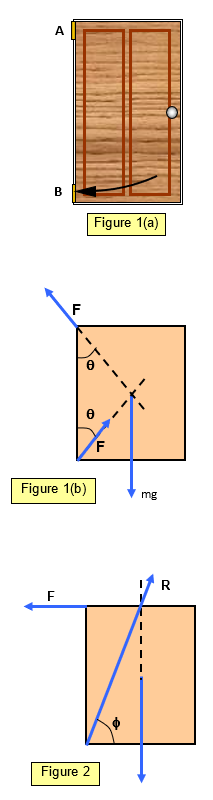# Vectors in equilibrium - The DoorThe forces in the hinges of a door is a useful demonstration of three vectors in equilibrium.

Figure 1(a) shows a door and the forces on the door are shown in Figures 1(b). (For simplicity the door is shown as completely uniform)

The hinges (A and B) are symmetrical, placed at the corners of the door and each supports half the weight of the door. The forces (F) in each hinge are equal.

To understand in which direction the forces on the door we must appreciate the following:
(a) the three forces on the door are
(i) its weight (mg)
(ii) the force due to hinge A
(iii) the force due to hinge B
(b) what happens if one or other of the hinges are disconnected from the door
(i) if hinge A is disconnected from the door the door will tip downwards and away from the frame (direction a in Figure 1(a)
(ii) if hinge B is disconnected from the door the door will tip downwards and inwards towards the frame (direction b in Figure 1(a)
This means that the force of hinge A is upwards and towards the frame and the force of hinge B is upwards and away from the frame. (See diagram 1(b).)

Resolving the forces on the door vertically we have:

2F = cosθ = mg

In Figure 2 the force of the top hinge is taken to be horizontal and the bottom hinge must therefore bear the full vertical weight of the door.

mg = R sin φ

A VERSION IN WORD IS AVAILABLE ON THE SCHOOLPHYSICS USB